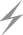Computer Aids for VLSI Design
Steven M. Rubin

Chapter 6: Dynamic Analysis Tools

This chapter was contributed by Robert W. Hon, Cadence Design Systems Inc.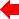Section 6 of 8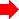## 6.6 Event-Driven Simulation

This section gives a brief example of how a gate-level event-driven simulation might operate on the cross-coupled NOR gates of Fig. 6.5.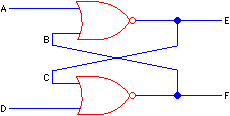FIGURE 6.5 Cross coupled NOR gates.

### 6.6.1 Example

In order to simulate the behavior of the circuit, a model of the behavior of each gate is needed. In simulating at the gate level, a truth table as shown in Fig. 6.6 is appropriate. Given the circuit and its functional behavior, only a test vector and a delay model are required to begin the simulation. Reasonable assumptions are that each gate requires a delay of one time unit, and that wires have a delay of zero time units. At time 0, all inputs and outputs are at logic value X. At some time t, input A is changed to logic value 1 and input D to 0.

The simulator then begins the algorithm given previously, which is repeated here:

1. Remove all events from the queue that occur at the next time t.
2. Note each input change at the appropriate node.
3. Calculate a new value for the output of each affected node.
4. Create an event for each node that is connected to the output of the affected node and insert it into the queue at time t + delay, where delay is determined by the propagation time through the node.

 In1 In2 Out 0 0 1 0 1 0 0 X X 1 0 0 1 1 0 1 X 0 X 0 X X 1 0 X X X
FIGURE 6.6 Truth table for NOR gate.

Applying the test vector places two events on the queue:

(A 1 t) (D 0 t)

In executing the algorithm, at time t the inputs to the top gate are 1X, which causes the creation of event (E 0 t+1). Since input C is connected to output E, the event (C 0 t+1) is also created. The inputs to the bottom gate are X0, which causes the creation of events (F X t+1) and (B X t+1). At the end of the first iteration of the algorithm, representing the circuit at time t, the event queue contains:

(E 0 t+1) (C 0 t+1) (F X t+1) (B X t+1)

Continuing at step 1, event (E 0 t+1) is removed. This change to output E requires no action, other than reporting the value by printing or plotting. In considering the event (B X t+1), it is observed that the event does not change the inputs to the top gate. Therefore no new events are created for that gate. (The simulator could have chosen to not check the previous value of the B input when considering the event [B X t+1], and entered the resulting events [E 0 t+2] [C 0 t+2] on the queue. This would not have affected the correctness of the simulation, but would have caused a considerable amount of needless computation.) The inputs to the bottom gate are now 00, so the events (F 1 t+2) (B 1 t+2) are added to the queue. At the end of time t+1, the queue contains:

(F 1 t+2) (B 1 t+2)

At time t+2, the inputs to the top gate are 11, so the events (E 0 t+3) (C 0 t+3) are added. At the end of time t+2, the queue contains:

(E 0 t+3) (C 0 t+3)

In the processing of these events no inputs are changed, so no new events need to be added to the queue, which is empty. At this point the algorithm terminates.

### 6.6.2 Discussion

This example focuses several important issues in simulation. A medium level of abstraction was chosen for this simulation. What are the implications of that choice? In simulating this circuit, about 10 events were processed. Even so, care was taken to avoid processing several events that had no affect on the circuit. In a circuit with several thousand gates, many hundreds of thousands of events might be processed. So it is important that the simulator is implemented efficiently, and that unnecessary events are not processed.

One way to avoid unnecessary event processing is to simulate with more abstract circuit elements. For example, we could replace the cross-coupled NOR gates with a two-input, two-output logic block the behavior of which is specified with a truth table. One line in the table indicates that inputs of 10 gives outputs of 01 after a delay of two time units. Simulating with this new logic block would result in only four events being processed (two for the test vector and two output events). This gives a faster simulation, but some information has been lost.

The NOR-gate simulation showed that the outputs actually settled independently: E changed to 0 at time t + 1, whereas F did not change to 1 until time t + 2. This information might have important implications, depending on the rest of the circuit, yet was not available when simulating with the more abstract circuit. The independent settling of the outputs is caused by the inclusion of feedback in the circuit, which is a common circuit-design technique. Feedback can cause many subtle timing problems, including race conditions and oscillations. As just demonstrated, the improper or unlucky choice of abstraction level can mask such problems.

Similarly, the NOR-gate simulation cannot model some types of behavior. It is well known that the simultaneous changing of gate inputs can lead to metastable behavior. The simple models used in this simulation cannot detect such behavior. A more detailed timing simulation or circuit-level simulation would be likely to find this type of problem, at the expense of time.Previous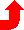Table of Contents NextSteven M. Rubin Static Free Software